# NCERT Solutions For Class 11 physics Gravitation## myCBSEguide App

CBSE, NCERT, JEE Main, NEET-UG, NDA, Exam Papers, Question Bank, NCERT Solutions, Exemplars, Revision Notes, Free Videos, MCQ Tests & more.

NCERT solutions for Class 11 physics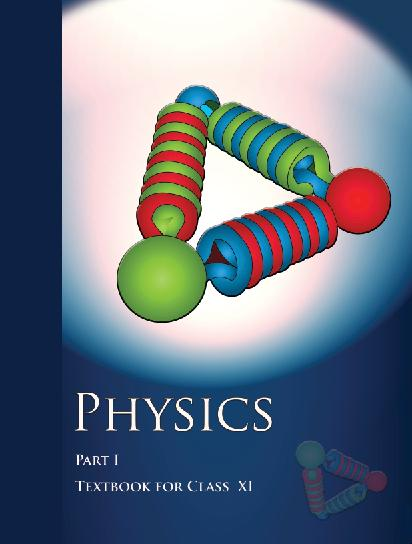## NCERT Class 11 Physics Chapter wise Solutions

1. Physical World
2. Units and Measurements
3. Motion in a Straight line
4. Motion in a Plane
5. Laws of Motion
6. Work, Energy and Power
7. System of Particles and Rotational motion
8. Gravitation
9. Mechanical Properties of Solids
10. Mechanical properties of fluids
11. Thermal Properties of matter
12. Thermodynamics
13. Kinetic Theory
14. Oscillations
15. Waves

## NCERT Solutions For Class 11 physics Gravitation

(a) You can shield a charge from electrical forces by putting it inside a hollow conductor. Can you shield a body from the gravitational influence of nearby matter by putting it inside a hollow sphere or by some other means?

(b) An astronaut inside a small space ship orbiting around the earth cannot detect gravity. If the space station orbiting around the earth has a large size, can he hope to detect gravity?

(c) If you compare the gravitational force on the earth due to the sun to that due to the moon, you would find that the Sun’s pull is greater than the moon’s pull. (You can check this yourself using the data available in the succeeding exercises). However, the tidal effect of the moon’s pull is greater than the tidal effect of sun. Why?

2. Choose the correct alternative:

(a) Acceleration due to gravity increases/decreases with increasing altitude.

(b) Acceleration due to gravity increases/decreases with increasing depth. (assume the earth to be a sphere of uniform density).

(c) Acceleration due to gravity is independent of mass of the earth/mass of the body.

(d) The formula –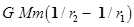is more/less accurate than the formula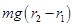for the difference of potential energy between two points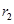and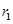distance away from the centre of the earth.

3. Suppose there existed a planet that went around the sun twice as fast as the earth. What would be its orbital size as compared to that of the earth?

4. Io, one of the satellites of Jupiter, has an orbital period of 1.769 days and the radius of the orbit is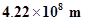. Show that the mass of Jupiter is about one-thousandth that of the sun.

5. Let us assume that our galaxy consists of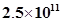stars each of one solar mass. How long will a star at a distance of 50,000 ly from the galactic centre take to complete one revolution? Take the diameter of the Milky Way to be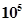ly.

6. Choose the correct alternative:

(a) If the zero of potential energy is at infinity, the total energy of an orbiting satellite is negative of its kinetic/potential energy.

(b) The energy required to launch an orbiting satellite out of earth’s gravitational influence is more/less than the energy required to project a stationary object at the same height (as the satellite) out of earth’s influence.

7. Does the escape speed of a body from the earth depend on

(a) the mass of the body,

(b) the location from where it is projected,

(c) the direction of projection,

(d) the height of the location from where the body is launched?

8. A comet orbits the Sun in a highly elliptical orbit. Does the comet have a constant (a) linear speed, (b) angular speed, (c) angular momentum, (d) kinetic energy, (e) potential energy, (f) total energy throughout its orbit? Neglect any mass loss of the comet when it comes very close to the Sun.

9. Which of the following symptoms is likely to afflict an astronaut in space (a) swollen feet, (b) swollen face, (c) headache, (d) orientational problem?

10. Choose the correct answer from among the given ones:

The gravitational intensity at the centre of a hemispherical shell of uniform mass density has the direction indicated by the arrow (see Fig 8.12) (i) a, (ii) b, (iii) c, (iv) O.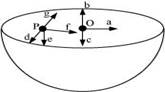These are first 10 questions only. To view and download complete NCERT Solution

the best CBSE App from google play store now or login to our Student Dashboard.

## CBSE app for Class 11

To download NCERT Solutions for class 11 Physics, Chemistry, Biology, History, Political Science, Economics, Geography, Computer Science, Home Science, Accountancy, Business Studies and Home Science; do check myCBSEguide app or website. myCBSEguide provides sample papers with solution, test papers for chapter-wise practice, NCERT solutions, NCERT Exemplar solutions, quick revision notes for ready reference, CBSE guess papers and CBSE important question papers. Sample Paper all are made available through the best app for CBSE students and myCBSEguide website### 1 thought on “NCERT Solutions For Class 11 physics Gravitation”

1. thanks for the soppourt online